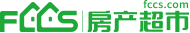【摘要】

（1）方便顾客购物

a、交通便利，是过往乘客的集中地段，人群流动性强，流动量大。如果是几个车站交汇点，则该地段的商业价值更高。超市开业之地如选择在这类地区就能给顾客提供便利购物的条件。
b、靠近人群聚集的场所，可方便顾客随机购物，如影剧院、商业街、公园名胜、娱乐、旅游地区等，这些地方可以使顾客享受到购物、休闲、娱乐、旅游等多种服务的便利，是超市开业的最佳地点选择。但此种地段属经商的黄金之地，寸土寸金，地价高费用大，竞争性也强。因而虽然商业效益好，但并非适合所有超市经营，一般只适合大型超市或有鲜明个性的专业商店的发展。
c、人口居住稠密区或机关单位集中的地区。由于这类地段人口密度大，且距离较近，顾客购物省时省力比较方便。商店地址如选在这类地段，会对顾客有较大吸引力，很容易培养忠实消费群。
d、符合客流规律和流向的人群集散地段。这类地段适应类地段适应顾客的生活习惯，自然形成“市场”，所以能够进入超市购物的顾客人数多，客流量大。
（2）有利于获取最大的经济效益

1、矩阵 为准则层的各个元素对目标的比较矩阵；
2、矩阵 为子准则层的各个元素对准则 的比较矩阵；
3、矩阵 为相对权重向量；
4、 为一致性比率；
5、 为一致性指标；
6、 为平均随机一致性指标；
7、AA*w1为组合权重；

（1） 新建的超市可以容纳所有到来的顾客，可以满足所有人的需求；
（2）各个区居民平均分布于各区；
（3）居民出行方式选择公交汽车，而公交汽车站点设立在各个主干道的交叉处；居民在主干道交接点处换乘汽车的时间忽略不计；
（4）人们只选择距离自己位置最近的超市进行购物消费。

1．模型的建立

1.1 构建评估指标结构模型

1.2 建立成对比矩阵

1.3 计算权重向量并做一致性检验

2.模型的求解
2.1比较矩阵的建立

2.2计算权重向量并一致性检验

 戴朝寿，孙世良.数学建模简明教程[M].第1版.北京：高等教育出版社，2007.
 胡良剑，孙晓君.MATLAB数学实验[M].第1版.北京：高等教育出版社，2006.
 白雪.基于层次分析法的大型综合超市选址分析.[J].中国商贸，2011（18）:177.
徐从桉.世界五百强超市的选址规划和设计—商务风水案例[M].第一版.北京:北京高教音像出版社，2006.
秦秋香.浅谈连锁超市选址的商圈分析方法.[J].商场现代化，2008(33).
方虹.跨国零售超市在华发展战略与策略研究.[M].第一版.北京：中国社会科学出版社，2004.

A=[1 1/3 1/2 3 ; 3 1 3/2 9; 2 2/3 1 3; 1/3 1/9 1/3 1];
[v,d]=eig(A);
eigenvalu=diag(d);
lamda=max(eigenvalue);
cil=(lamda-4)/3;
crl=cil/1.26;
w1=v(:,1)/sum(v(:,1))

w1 =

0.1638
0.4914
0.2788
0.0660

A=[1 1/3 1/2 3 ; 3 1 3/2 9; 2 2/3 1 3; 1/3 1/9 1/3 1];
w1=[0.1638 ; 0.4914  ; 0.2788  ; 0.0660];
A*w1

ans =

0.6650
1.9950
1.1320
0.2681

B1=[1 3/2 3;2/3 1 2;1/3 1/2 1];
[v,d]=eig(B1);
eigenvalu=diag(d);
lamda=max(eigenvalue);
w2=v(:,1)/sum(v(:,1))

w2 =

0.5000
0.3333
0.1667

B1=[1 3/2 3;2/3 1 2;1/3 1/2 1];
w2 =[ 0.5000;0.3333;0.1667];
B1*w2

ans =

1.5000
1.0000
0.5000

B2=[1 2 1/3;1/2 1 1/4;3 4 1];
[v,d]=eig(B2);
eigenvalu=diag(d);
lamda=max(eigenvalue);
w3=v(:,1)/sum(v(:,1))

w3 =

0.2385
0.1365
0.6250

B2=[1 2 1/3;1/2 1 1/4;3 4 1];
w3 =[0.2385;0.1365;0.6250];
B2*w3

ans =

0.7198
0.4120
1.8865

B3=[1 3 1/2;1/3 1 1/6;2 6 1];
[v,d]=eig(B3);
eigenvalu=diag(d);
lamda=max(eigenvalue);
w4=v(:,1)/sum(v(:,1))

w4 =

0.3000
0.1000
0.6000

B3=[1 3 1/2;1/3 1 1/6;2 6 1];
w4 =[0.3000;
0.1000;
0.6000];

B3*w4

ans =

0.9000
0.3000
1.8000

B4=[1  1/2; 2 1];
[v,d]=eig(B4);
eigenvalu=diag(d);
lamda=max(eigenvalue);
w5=v(:,1)/sum(v(:,1))

w5 =

0.3333
0.6667

B4=[1  1/2; 2 1];
w5 =[0.3333;
0.6667];
B4*w5

ans =

0.6666
1.3333

AA=[0.5 0.2385  0.3  0.3333;0.3333 0.1365 0.1  0.6667 ;0.1667 0.625 0.6 0;0 0 0 0];
w1=[0.1638;
0.4914;
0.2788;
0.0660];
AA*w1

ans =

0.3047
0.1936
0.5017
0

-->
 关于我们 ┊网站律师 ┊联系我们 ┊诚聘英才 ┊友情链接 ┊M版找房 ┊每日资讯 ┊免责声明 ┊用户协议 ┊使用帮助 ┊手机客户端

 电子公告（BBS）业务许可证  广播电视节目制作经营许可证(浙)字第1038号  商业特许经营备案号：1330402000900005